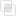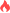python Axes3D绘制3D图形

Python Axes3D2021-08-25 14:01:381274浏览 · 0收藏 · 0评论1、绘制3D坐标的函数Axes3D。

2、X轴-2到2之间，Y轴-2到2之间。

3、计算sin函数赋值为Z坐标。

4、给三个坐标轴注明。

# -*- coding: utf-8 -*-
#By:Eastmount CSDN
from matplotlib import pyplot as plt
import numpy as np
from mpl_toolkits.mplot3d import Axes3D  # 绘制3D坐标的函数

fig = plt.figure()               #创建一个绘图对象
ax = Axes3D(fig)                 #用这个绘图对象创建一个Axes对象
X = np.arange(-2, 2, 0.25)       #X轴-2到2之间
Y = np.arange(-2, 2, 0.25)       #Y轴-2到2之间
print(Y)

X, Y = np.meshgrid(X, Y)         #用两个坐标轴上的点在平面上画格
R = np.sqrt(X**2 + Y**2)         #X和Y的平方和开根号
Z = np.sin(R)                    #计算sin函数赋值为Z坐标

#具体函数方法可用 help(function) 查看，如：help(ax.plot_surface)
ax.plot_surface(X, Y, Z, rstride=1, cstride=1, cmap='rainbow')

#给三个坐标轴注明
ax.set_xlabel('x label', color='r')
ax.set_ylabel('y label', color='g')
ax.set_zlabel('z label', color='b')

plt.show()python如何模拟用户自动打卡python逻辑取反的实现python Pytest有什么特点python如何打印矩阵Python Modules是什么意思python三种导入模块的方式python查找计算函数的整理python有哪些转换字符串的函数python填充压缩的函数总结python分割拼接函数的介绍python判断字符串函数的归纳python如何实现图像等比缩放python setup和teardown的使用python绘制散点图的两种方法python用plt.pie绘制饼图js事件委托如何理解8223

js中hasOwnProperty的属性用法7368